## Journal of Biomedical Imaging and Bioengineering

All submissions of the EM system will be redirected to Online Manuscript Submission System. Authors are requested to submit articles directly to Online Manuscript Submission System of respective journal.

Research Article - Journal of Biomedical Imaging and Bioengineering (2019) Volume 3, Issue 1

## Error elimination algorithm for semi-automated Hessian matrix candidate selection specially for retinal images with exudates to detect diabetic retinopathy.

Shreya Singh Chauhan*, Awadhesh Gupta, Rekha Gupta

*Corresponding Author:
Shreya Singh Chauhan
Madhav Institute of Technology and Science
Gwalior
E-mail:
[email protected]

Accepted date: May 10, 2019

Visit for more related articles at Journal of Biomedical Imaging and Bioengineering

### Abstract

Image processing has been used to detect Diabetic Retinopathy (DR) by using computer based algorithms. Diabetic Retinopathy may leads to vision loss occurs due to retinal disorders. There are many algorithms proposed by researchers in which one of the popular algorithms is SHCS. But SHCS algorithm didn’t work better with retinal images with exudates for detection of Microaneurysms (MA) &Hemorrhages (HMA). The proposed error elimination algorithm is very effective in removing false negative in the retinal image.

## Keywords

Diabetic Retinopathy (DR), Microaneurysms (MAs), Hemorrhages (HMAs), Semi-automated Hessianbased Candidate Selection (SHCS) algorithm, Median Filter (MF), Red, Green & Blue (RGB), Computer Aided Diagnosis (CAD), Low Pass Filter (LPF), Exudates

## Introduction

Diabetic patients are more prone to vision loss that happens due to retinal disorder. Microaneurysms (MAs) and hemorrhages (HMAs) appears in the retinal image of eyes which indicates that the patient is suffered from Diabetic Retinopathy. Since DR does not show any distinctive symptoms therefore regular check-ups from a specialized ophthalmologists is required. But due to few available specialist, the cost of treatment increases. In order to solve this problem, systems are created which are of cheap cost &has a CAD. HMAs & MAs are the foremost clinical signs which shows that person is suffering from DR (diabetic retinopathy). MAs are minute blood-filled bulges. MAs are presents in the artery walls but HMAs destroy the retentive tissue at the back wall of human eyes; HMA appears as a spots of red colour which looks slightly larger than MA as shown in Figure 1 . DR issue happen because of diabetes and it may results in irreversible type of blindness in the patient. Early detection of DR requires accurate detection of MA and computerized diagnosis insures reliable and accurate detection of MA's .

In eyes, exudates are formed in retinal image due to the damage in retinal blood vessels. Exudates are randomly spread over the retina and appear as yellow-white patches of varying sizes and shapes which are basically a broken vessels leaks the lipids and proteins around the retina . Development of MA, HMA & exudates in the eye determine the intensity of disease with which a person is ill [4,5]. The movement of exudates towards the macular region of the eye shows the symptoms of total loss of vision .

Semi-automated Hessian-based candidate selection (SHCS) algorithm very is popular among other algorithms. After applying SHCS on retinal images with exudates, it is found that, the algorithm gives lots of false negative. In order to solve it, error elimination algorithm has been proposed. The proposed has been successfully eliminated false negative around exudates. SHCS algorithm followed by proposed algorithm gives much better result in retinal images with exudates to find MA & HMA as compared to SHCS algorithm alone.

## Method

SHCS algorithm

In Semi-automated DR detection algorithm, first image preprocessing is applied in which green-channel image is taken out of the input retinal coloured image as it is analysed by researchers that the contrast of the HMA &MA appears high in the green component of the image. In order to reduce the noise in the image, LPF is applied on it .

In the next step, eigen value analysis which is based on Hessian matrix is performed to find MA & HMA in the eye. Hessian matrix can be taken as a matrix of 2nd order partial differentiation derived functions. As the intensity curve surface of input image is approximated by the partial derivative function then the Hessian matrix can be shown as

Hes (x,y)=Vxx(x,y) Vxy(x,y)

Vy(x,) Vyy(x,) (1)

Vxx (x,y)=GAUxx (x,y)*Img (x,y) (2)

Vxx (x,y)=GAUxx (x,y)*Img (x,y) (3)

Vxx (x,y)=GAUxx (x,y)*Img (x,y) (4)

Vxx (x,y)=GAUxx (x,y)*Img (x,y) (5)

Here * represents convolution operator, Img (x,y) is the preprocessed image, GAUxx (x,y),GAUxy(x,y), GAUyx (x,y), GAUyy (x,y) are the 2nd order partial derivative functions of the Gaussian function in all direction . Where Gaussian functioncan be equated as,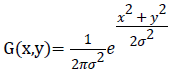(6)

Where σ is the parameter to determine the scale of the Gaussian function.

In order to find dark blob like structure, green channel fundus image is inserted in the Hessian operator and if they exhibit strong derivatives in the two orthogonal directions then it is considered as detected . Eigenvalues are calculated from the obtained Hessian matrix. With pre-defined eigen values, model it belongs to and resulting behaviour of the eigenvalues, the structure can be searched by proper analysing the voxel. The region of interest can be determined by comparing Eigen values λ1 & λ2 with the pre-defined threshold values. Table 1 below summarizes the relation between λi and their respective structure orientation in the image .

λ 1 λ 2 Structure orientation
Low Low Noise
Low Low light color sheet
Low Low Dark color sheet
Low High- Light color tube
Low High+ dark color tube
High- High- Light color blob
High+ High+ Dark color blob

Table 1. Hessian matrix eigen values threshold (λ1 & λ2) determining image structure& orientation.

It is possible a false detection because of the fixed threshold value because eigen values of different MA may different at different part of the eye [8,9].

As in our work, it is required to detect dark blob like structure therefore threshold is selected as λ1=1 & λ2=1.

Proposed algorithm

Firstly Semi-automated DR detection is applied on the retinal image with exudates. The results of SHCS has lots of errors due to the presence of exudates, therefore this image is passed through proposed error elimination algorithm as shown in flowchart Figure 2.

If F (i,j) is the detected MA or HMA in the output of the SHCS algorithm then output of error elimination algorithm is given by,

F(i,j)={true positive, if condition satisfied error, otherwise} (7)

Where condition is,

Fp (i-1,j)<T OR Fp (i+1,j)<T OR Fp (i,j+1)<T ORFp (i,j-1)<T

Here,

Fp=neighboring pixels around Fp (i,j)

T=threshold value, taken as 70 here.

It means if all the neighboring pixels are less than threshold value T then it means they all are dark in colour and then are true positive else if anyone of the neighboring pixel is lighter in colour then it means it is a part of exudate which is wrongly detected and thus discarded by proposed algorithm.

## Results and Discussion

Below are the simulation results which are simulated in MATLAB. For experimentation, images are taken from online retinopathy challenge database . The performance parameter to determine the quality of work is TPR (true positive rate). TPR can be defined as a number of correct positive results obtained during the test from all the available positive samples under consideration. True positive rate can be equated as,

TPR (True Positive Rate)=(TP/TP+FN)*100 (9)

Figure 3 shows the results out of SHCS algorithm where (A) contains the final output with HMA & MA, (B) shows the segmentation output and (C) shows the zoom version of exudate part of the image to compare it with proposed work.

Similarly, Figure 4 shows the results out of proposed algorithm where (A) contains the final output with HMA & MA, (B) shows the segmentation output and (C) shows the zoom version of exudate part of the image to compare it with previous work.

Table 2 shows the output images of both previous and proposed work. Images have been zoomed for comparison of the results. It can be clearly seen that proposed algorithm is successful in removing error or false detections.

S.No. Previous work output Proposed work output
1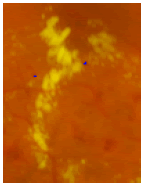2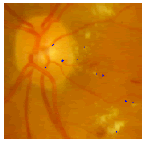3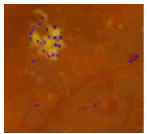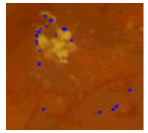4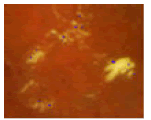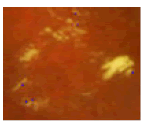5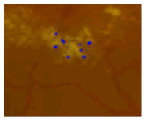Table 2. Results of previous and proposed work.

Table 3 contains the data regarding TPR rate for both previous and proposed work, which has been calculated by counting TP (True Positive) and FN (False Negative). Figure 4 shows the bar graph for Table 2, which clearly shows that detection by proposed algorithm for all the images are better in proposed work than the results of previous work.

S.No. Previous work Proposed work
TP FN TPR TP FN TPR
1 23 58 28.75 23 8 74.19
2 7 23 23.33 8 2 80
3 26 133 16.35 57 72 44.14
4 2 14 12.5 3 6 33.33
5 4 36 10 7 19 26.92

Table 3. Results of previous and proposed work in tabular form.

## Conclusion

SHCS algorithms based on analysis of eigen values of Hessian matrix is popular in detection of MA, HMA and Exudates. But for the retinal image with exudates it wrongly detects MA and HMA around exudates. The proposed error elimination algorithm is effective to improve the results of the SHCS by removing false detections. TPR for retinal image with MA & HMA is calculates as 0.28 by using previous work algorithm while for proposed work it is 0.74 for the image with exudates. Due to the presence of exudates, false negative of the image increases which is successfully removed by our proposed error elimination algorithm.

Get the App